The SYSLIN Procedure

Estimation Methods

Subsections:

A brief description of the methods used by the SYSLIN procedure follows. For more information about these methods, see the references at the end of this chapter.

There are two fundamental methods of estimation for simultaneous equations: least squares and maximum likelihood. There are two approaches within each of these categories: single equation methods (also referred to as limited information methods) and system methods (also referred to as full information methods). System methods take into account cross-equation correlations of the disturbances in estimating parameters, while single equation methods do not.

OLS, 2SLS, MELO, K-class, SUR, ITSUR, 3SLS, and IT3SLS use the least squares method; LIML and FIML use the maximum likelihood method.

OLS, 2SLS, MELO, K-class, and LIML are single equation methods. The system methods are SUR, ITSUR, 3SLS, IT3SLS, and FIML.

Single Equation Estimation Methods

Single equation methods do not take into account correlations of errors across equations. As a result, these estimators are not asymptotically efficient compared to full information methods; however, there are instances in which they may be preferred. (See the section Choosing a Method for Simultaneous Equations for details.)

Letbe the dependent endogenous variable in equation i, and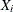and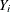be the matrices of exogenous and endogenous variables appearing as regressors in the same equation.

The 2SLS method owes its name to the fact that, in a first stage, the instrumental variables are used as regressors to obtain a projected value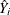that is uncorrelated with the residual in equation i. In a second stage,replaceson the right-hand side to obtain consistent least squares estimators.

Normally, the predetermined variables of the system are used as the instruments. It is possible to use variables other than predetermined variables from your system as instruments; however, the estimation might not be as efficient. For consistent estimates, the instruments must be uncorrelated with the residual and correlated with the endogenous variables.

The LIML method results in consistent estimates that are equal to the 2SLS estimates when an equation is exactly identified. LIML can be viewed as a least-variance ratio estimation or as a maximum likelihood estimation. LIML involves minimizing the ratio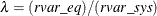, where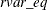is the residual variance associated with regressing the weighted endogenous variables on all predetermined variables that appear in that equation, and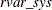is the residual variance associated with regressing weighted endogenous variables on all predetermined variables in the system.

The MELO method computes the minimum expected loss estimator. MELO estimators "minimize the posterior expectation of generalized quadratic loss functions for structural coefficients of linear structural models" (Judge et al., 1985, p. 635).

K-class estimators are a class of estimators that depends on a user-specified parameter k. A k value less than 1 is recommended but not required. The parameter k can be deterministic or stochastic, but its probability limit must equal 1 for consistent parameter estimates. When all the predetermined variables are listed as instruments, they include all the other single equation estimators supported by PROC SYSLIN. The instance when some of the predetermined variables are not listed among the instruments is not supported by PROC SYSLIN for the general K-class estimation. However, it is supported for the other methods.

For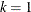, the K-class estimator is the 2SLS estimator, while for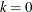, the K-class estimator is the OLS estimator. The K-class interpretation of LIML is that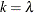. Note that k is stochastic in the LIML method, unlike for OLS and 2SLS.

MELO is a Bayesian K-class estimator. It yields estimates that can be expressed as a matrix-weighted average of the OLS and 2SLS estimates. MELO estimators have finite second moments and hence finite risk. Other frequently used K-class estimators might not have finite moments under some commonly encountered circumstances, and hence there can be infinite risk relative to quadratic and other loss functions.

One way of comparing K-class estimators is to note that when k =1, the correlation between regressor and the residual is completely corrected for. In all other cases, it is only partially corrected for.

See Computational Details for more details about K-class estimators.

SUR and 3SLS Estimation Methods

SUR might improve the efficiency of parameter estimates when there is contemporaneous correlation of errors across equations. In practice, the contemporaneous correlation matrix is estimated using OLS residuals. Under two sets of circumstances, SUR parameter estimates are the same as those produced by OLS: when there is no contemporaneous correlation of errors across equations (the estimate of the contemporaneous correlation matrix is diagonal) and when the independent variables are the same across equations.

Theoretically, SUR parameter estimates are always at least as efficient as OLS in large samples, provided that your equations are correctly specified. However, in small samples the need to estimate the covariance matrix from the OLS residuals increases the sampling variability of the SUR estimates. This effect can cause SUR to be less efficient than OLS. If the sample size is small and the cross-equation correlations are small, then OLS is preferred to SUR. The consequences of specification error are also more serious with SUR than with OLS.

The 3SLS method combines the ideas of the 2SLS and SUR methods. Like 2SLS, the 3SLS method uses
instead of
for endogenous regressors, which results in consistent estimates. Like SUR, the 3SLS method takes the cross-equation error correlations into account to improve large sample efficiency. For 3SLS, the 2SLS residuals are used to estimate the cross-equation error covariance matrix.

The SUR and 3SLS methods can be iterated by recomputing the estimate of the cross-equation covariance matrix from the SUR or 3SLS residuals and then computing new SUR or 3SLS estimates based on this updated covariance matrix estimate. Continuing this iteration until convergence produces ITSUR or IT3SLS estimates.

FIML Estimation Method

The FIML estimator is a system generalization of the LIML estimator. The FIML method involves minimizing the determinant of the covariance matrix associated with residuals of the reduced form of the equation system. From a maximum likelihood standpoint, the LIML method involves assuming that the errors are normally distributed and then maximizing the likelihood function subject to restrictions on a particular equation. FIML is similar, except that the likelihood function is maximized subject to restrictions on all of the parameters in the model, not just those in the equation being estimated.

Note: The RESTRICT, SRESTRICT, TEST, and STEST statements are not supported when the FIML method is used.

Choosing a Method for Simultaneous Equations

A number of factors should be taken into account in choosing an estimation method. Although system methods are asymptotically most efficient in the absence of specification error, system methods are more sensitive to specification error than single equation methods.

In practice, models are never perfectly specified. It is a matter of judgment whether the misspecification is serious enough to warrant avoidance of system methods.

Another factor to consider is sample size. With small samples, 2SLS might be preferred to 3SLS. In general, it is difficult to say much about the small sample properties of K-class estimators because the results depend on the regressors used.

LIML and FIML are invariant to the normalization rule imposed but are computationally more expensive than 2SLS or 3SLS.

If the reason for contemporaneous correlation among errors across equations is a common, omitted variable, it is not necessarily best to apply SUR. SUR parameter estimates are more sensitive to specification error than OLS. OLS might produce better parameter estimates under these circumstances. SUR estimates are also affected by the sampling variation of the error covariance matrix. There is some evidence from Monte Carlo studies that SUR is less efficient than OLS in small samples.